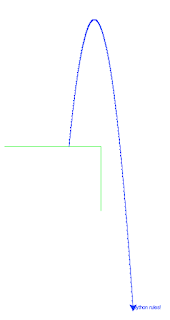## Saturday, 23 December 2017

### Gravity Simulations for Year 6/7 Students

This note discusses Python programming for year 6/7 students at a local primary school.  The activity was part of a STEM program coordinated by CSIRO.

Python provides an excellent option for introducing programming in schools and has been widely adopted in the UK.  After discussions with the class teacher, we decided to use Python within the area of Space Science.  In particular the lessons aimed to introduce the elements of programming in a Python development environment using "Turtle Graphics", with a focus on simulating the motion of heaven bodies subject to gravity.

Primary students can make very good progress with programming concepts and are keen to learn.   However the motion of bodies subject to acceleration is normally a high-school topic.  The examples below aim to show that using first-order equations can put this topic within reach of primary students.  We need the following "maths":
• distance travelled = velocity * time interval
• change in velocity = acceleration * time interval
• force = mass * acceleration

The last equation, which is Newton's famous second law, is not actually used in the examples below but provides a great way to talk about the concepts involved, including large rockets that can provide huge thrust forces!   The second equation is basically the definition of acceleration, which might be a novel concept for younger programmers.  We are all familiar with the first equation and strictly speaking this only applies when the velocity is fixed.  Our simulation approach is to take many small time steps and calculate the object's position and velocity at each step.  As long as the velocity is changing "smoothly" this simulation approach gives a good approximation to the formulas normally used (eg s=ut + at^2/2 etc).Graphics output from gravity_order1.py
Anyway, two examples are shown below using this approach.  Note that to further simplify the problems we assume that the force of gravity only acts vertically and affects vertical motion, with no acceleration on the horizontal direction.

In the first example a ball is thrown upwards and falls due to gravity.  The graphical output is shown on the left.   The code (below) is very simple:  after some initialisation statements, a loop is used to evaluate the ball's vertical velocity and position at each time step.From lander.py

As a second example consider a lunar lander simulation, where the thruster on the lander can be toggled on and off by pressing the 'up' key. A sample output is shown on the right.  Initially the lander has zero vertical velocity and a small horizontal velocity.  It starts to accelerate towards the lunar surface due to the moon's gravity, as shown by the increasing distance between the black dots at each time step.  When the thruster is turned on, the position dots change to red.  For simplicity, we assume in the code (below) that the thruster causes an acceleration of equal magnitude, but upwards. Hence the rate of descent decreases until the thruster is turned off, after which position is shown in blue.  By toggling the thruster, the lander can be brought gently to the surface.  This takes a little practice!

It would have been nice to retain a simple simulation loop like the first example, but include a 'key-pressed' check for thruster control.  That doesn't seem possible in this environment, so the code for this example uses an 'event-driven' programming model.  The position and velocity calculations reside in function 'tloop' and the thruster is called from a key-press handling function.